# GSEB Solutions Class 11 Chemistry Chapter 10 The s-Block Elements

Gujarat Board GSEB Textbook Solutions Class 11 Chemistry Chapter 10 The s-Block Elements Textbook Questions and Answers.

## Gujarat Board Textbook Solutions Class 11 Chemistry Chapter 10 The s-Block Elements

### GSEB Class 11 Chemistry The s-Block Elements Text Book Questions and AnswersQuestion 1.
What are the common physical and chemical features of alkali metals?
Alkali metals constitute group-I of the s-block of the periodic table. The alkali metals are characterised by the presence of one s-electron in the valence shell of their atoms.

(a)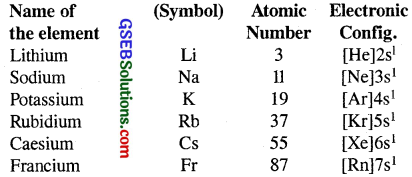(b) Atomic and ionic size : The alkali metals atoms have the largest sizes in a particular period in the periodic table. Their monovalent ions M+ are smaller than the parent atom.

(c) Ionisation enthalpy : The ionisation enthalpies of the alkali metals are considerably low.

Physical Properties :
(i) Physical state : All elements are silvery white, soft and light metals.

(ii) Density : Their densities are quite low and increase from lithium to caesium. However potassium is lighter than sodium.

(iii) Melting and Boiling points : They are low because of the weak bonding in the crystal lattices of the metals.

(iv) On account of their low ionisation energies, these metal atoms have great tendency to lose ns1 electron and form positive ions. M → M+ + e

Chemical Properties :
(i) Action of air : The alkali metals are tarnished in air due to the formation of oxide at their surface and hence they are stored in k rosene oil.
4Li + O2 → 2Li2O
Sodium forms sodium peroxide (Na2O2) and other alkali metals form superoxides [MO2 where M = K, Rb, si]

(ii) Action of water: Alkali metals react readily and vigorously with water forming hydroxides with the liberation of hydrogen.
2M + 2H2O → 2MOH + H2 [M = Li, Na, K, Rb, Cs]

(iii) Action of Hydrogen:
2M + H2 → MH [M = Li, Na, K etc.] (Ionic hydride)

(iv) Action with halogens:
2M + X2 → 2MX (Metal halide)

(v) Solubility in liquid ammonia : All alkali metals are soluble in liquid ammonia solutions and are blue in dilute metal-ammonia solutions. Such solutions are reducing in nature.

(vi) Reducing properties : The tendency to lose electrons is an important property of these metals. Thus all these metals act as strong reducing agents.
Na → Na+ + e
Among these, metal lithium is the strongest reducing agent.

(vii) Formation of alloys : The alkali metal form alloys amongst themselves as well as with other metals. The alkali metals dissolve readily in mercury forming amalgams. The process is highly exothermic.Question 2.
Discuss the general characteristics and gradation in properties of alkaline earth metals.
General characteristics of the alkaline earth metal :
Group 2 of the periodic table comprises the elements beryllium, magnesium, calcium, strontium, barium and radium. These (except beryllium) are known as the alkaline earth metals.

(i) Atomic Properties :
(a) Electronic configuration : They are characterised by the presence of two electrons in the valence shells of their atoms (ns2). These two electrons are present in the s-subshell of the valence shell Electronic configuration of the metal M – (Noble gas)ns²

(b) Atomic and ionic size : The atomic and ionic radii of these metals are smaller than those of alkali metals and from top to bottom, the atomic and ionic sizes increase.
Be < Mg < Ca < Sr < Ba
Be2+ < Mg2+ < Ca2+ < Sr2+ < Ba2+
But Mg2+ < Mg, Ca2+ < Ca & so on.

(c) Ionisation Enthalpies : The first ionisation enthalpies of the alkaline earth metals are higher than those of alkali metals, because of increase in nuclear charge. The 2nd I.Es of alkaline earth metals are smaller than those of the corresponding alkali metals. From top of bottom. I. Es decrease with the addition of one orbit each time.

(ii) Physical Properties of Alkaline Earth Metals –
(a) Physical appearance : These metals are generally silvery white, lustrous and relatively soft, but harder than the alkali metals. Be & Mg are somewhat greyish.

(b) Melting and boiling point: The fairly higher melting and boiling points of the alkaline earth metal compared to these of the corresponding alkali metal are attributed to their smaller sizes and two valence electrons. The trend is, however, not regular. M.Pts & B.Pts are the highest in the group.

(c) Flame colourations : Chlorides of alkaline earth metal (except that of Be and Mg) produce characteristic colour to the flame due to easy excitation of electron to higher energy levels.

(d) Densities : Leaving Mg and Ca which are.lighter, densities increase from Be < Sr < Ba < Ra.

(iii) Chemical Properties Of Alkaline Earth Metals –
The alkaline earth metals are less reactive than the corresponding alkali metal. The reactivity of these elements increases on going down the group.

(i) Reactivity towards air and water : Be and Mg are kinetically inert to O2 and H2O because of the formation of oxide film on their surface.

However Be in the powdered form burns brilliantly on ignition to give BeO and Be3N2.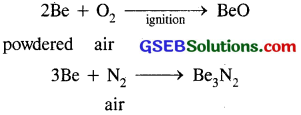Similarly Mg which is more electro +ve than Be burns with dazzling brilliance in air to give MgO and Mg3 N2. Ca, Sr, Ba are readily attacked by air to form the oxide and nitride.

(ii) Reactivity towards the halogens : All the alkaline earth metals react with halogens at elevated temperature forming halides.
M + X2 → MX2 [X = F, Cl, Br, I]

(iii) Reactivity towards hydrogen : Except Be, all other alkaline earth metals combine with H2 upon heating to form hydrids.
Ca + H2 → CaH2

(iv) Reactivity towards acids : The alkaline earth metal readily react with acids liberating H2 gas.
Mg(s) + 2HCl(aq) → MgCl2(aq) + H2

(v) Reducing nature: Like alkali metals, the alkaline earth metals are strong reducing agents, but their reducing power is less than that of alkali metals. Generally reducing character increases from top to bottom.

(vi) Solutions in liquid ammonia : Like alkali metals, the alkaline earth metals dissolve in liquid ammonia to give deep blue black solutions.
M + (x + y) NH3 → M(NH3)2+x + 2e (NH3)y.Question 3.
The loosely held s-electron in the outermost valence shell of atoms of these metal makes them the most electropositive metals which readily give M+ ions. Hence they are not found in free state in nature.

Question 4.
Find out the oxidation state of sodium in Na2O2.
Let the ox. no. of sodium (Na) = x
It is a peroxide
∴ Ox. no. of O = -1
∴ 2x + 2( -1) = 9
2x – 2 = 0 2x = 2
x = +1
∴ Oxidation state of sodium = + 1.

Question 5.
Explain why is sodium less reactive than potassium?
Because ionisation enthalpy of sodium is higher than that of potassium. Therefore it will lose electrons less readily as compared to potassium. Hence it is less reactive than potassium.

Question 6.
Compare the alkali metals and alkaline earth metals with respect to
(i) ionisation enthalpy
(ii) basicity of oxides
(iii) solubility of hydroxides.
(i) Ionisation enthalpy : The first ionisation enthalpies of alkaline earth metals are higher than those of alkali metals because of increased nuclear charge. However 2nd ionisation enthalpies of alkaline earth metals are lower than those of corresponding alkali metals, i.e. 1st ionisation enthalpy of Be > Li
Mg > Na and so on.

(ii) Basicity of oxides : The oxides of alkaline earth metals a. less basic as compared to the corresponding oxides of alkali n. eta because of increased nuclear charge their ionisation enthalpie higher.

(iii) Solubilities of hydroxides : Alkaline earth are less soluble in water as compared to the alkali.Question 7.
In what ways lithium shows similarities to cesium in its chemical behaviour?
Lithium resembles magnesium mainly due to me Similarity in sizes of their atoms [Li = 152 pm, Mg = 160 pm] and ions [Li+ = 76 pm, Mg2+ = 72 pm].

The main points of similarity are :
(i) Both LiOH and Mg(OH)2 are weak bases.

(ii) Both form ionic nitrides in an atmosphere of N2.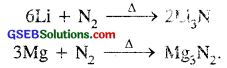(iii) The hydroxides and carbonates of both Li and Mg decompose upon heating to give their respective oxides.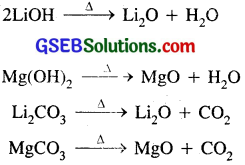(iv) Both lithium and magnesium do not form solid bicarbonates.

(v) Li2O and MgO do not combine with excess of oxygen to give peroxide or superoxides.

(vi) Both LiNO3 and Mg(NO3), decompose on heating producing nitrogen dioxide.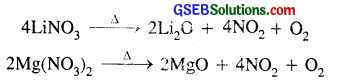(vii) The hydroxides, carbonates and fluorides of both lithium and magnesium are sparingly soluble in water.

(viii)Because of covalent character LiCl and MgCl2 are soluble in ethanol.

(ix) Both LiClO4 and Mg(ClO4)2 are highly soluble in ethanol.

(x) LiCl and MgCl2 are deliquescent and crystallise from aqueous solution as hydrates LiCl.2H2O and MgCl2.2H2O.

Question 8.
Explain why can alkali and alkaline earth metals not be obtained by chemical reduction methods?
(i) Alkali and alkaline earth metals are themselves strong reducing agents and hence cannot be extracted by reduction of their oxides and alkaline earth metals.

(ii) Alkali metals being highly electropositive cannot be displaced from the aqueous solutions of their salts by other metal.

Question 9.
Why are potassium and caesium, rather than lithium used in photo-electric cells?
Due to low ionisation enthalpy, potassium and caesium eject electrons when exposed to light. Lithium which has the highest ionisation enthalpy among the alkali metals cannot be used in photo-electric cells because it does not release electrons when exposed to light.Question 10.
When an alkali metal dissolves in liquid ammonia, the solution acquires different colours. Explain the reasons for this type colour change.
All alkali metals dissolve in liquid ammonia giving highly conducting deep blue solutions.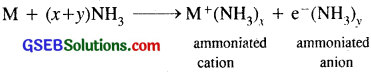(i) When ordinary light falls on these ammoniated electrons, they get excited and jump to higher energy levels by absorbing energy corresponding to red region of the visible light. As a result, transmitted light is blue which imparts blue colour to the solution.

(ii) Dilute solutions of alkali metals in liquid ammonia are dark blue in colour, but as the concentration increases above 3M, the colour changes to copper-bronze and the solutions acquire metallic lustre due to the formation of metal ion clusters:

Question 11.
Beryllium and magnesium do not give colour to flame whereas other alkaline earth metals do so why?
Chlorides of alkaline earth metals, except that of beryllium and magnesium produce characteristics colour of flame due to easy excitation of electrons higher energy levels. Be and Mg due to their small size, bind their electrons more strongly because their ionisation enthalpies are higher. Hence these require higher energy levels. Therefore, their electrons, do not get excited and do not impart any colour to the flame.

Question 12.
Discuss the various reactions that occur in the SOLVAY process.
Sodium carbonate (Na2CO3) is manufactured by SOLVAY process.
(i) Ammonium carbonate [(NH4)2CO3] is prepared by passing CO2 through a concentrated solution of sodium chloride [NaCl] saturated with ammonia (NH3).
2NH3 + H2O + CO2 → (NH4)2CO3

(ii) Ammonium carbonate further reacts with CO2 to produce ammonium bi-carbonate [NH4HCO3]

(iii) Ammonium bi-carbonate reacts with NaCI to form sodium bi-carbonate [NaHCO3]
NaCI + NH4HCO3 → NH4Cl + NaHCO3.

(iv) Sodium bicarbonate crystals separate. These are heated to give sodium carbonate (washing soda)
2NaHCO3 → Na2CO3 + CO2 + H2O

In this process NH3 is recovered when the solution containing NH4Cl is treated with Ca(OH)2. Calcium chloride (CaCl2) is obtained as a by-product.
2NH4Cl + Ca(OH)2 → 2NH3 + CaCl2 + H2.Question 13.
Potassium carbonate cannot be prepared by Solvay process why?
Potassium carbonate cannot be prepared by Solvay process, because potassium bicarbonate is to soluble to be precipitated by the addition of ammonium bicarbonate to a saturated solution of potassium chloride.

Question 14.
Why is Li2CO3 decomposed at a lower temperature whereas Na2CO3 at higher temperature?
Li2CO3 → Li2O + CO2
Li2CO3 is not stable and decomposes to form Li2O, because Li+ ion is small as compared to CO2-3 in the lattice of Li2CO3 and breaks apart to form a stable crystal lattice of smaller Li+ ions and smaller O2- ions in Li2O, whereas Na+ ions are larger than Li+ ions and forms a stable crystal lattice with larger CO2-3 ions. Therefore Li2CO3 decomposes to form a stable crystal lattice of Li2O, whereas Na2CO3 is quite stable.

Question 15.
Compare the solubility and thermal stability of the following compounds of the alkali metals with those of alkaline earth metals
(a) nitrates
(b) carbonates
(c) sulphates.
(a) Nitrates of alkali metals and alkaline earth metal.

(i) Nitrates of alkali metals are thermally not stable and decompose on heating to give MNO2 and O2 (except LiNO3) whereas nitrates of alkaline earth metals decompose on heating give their oxides, nitrogen dioxide and oxygen gas.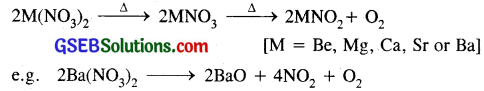(ii) Nitrates of alkali metals are highly soluble in water whereas alkaline earth metal nitrates are sparingly soluble and crystallise with six molecules of water.

(b) Carbonates of alkali metals and alkaline earth metals :

(i) Carbonate of alkali metal except Li are quite stable upto 1273 K do not decompose, whereas carbonates of alkaline earth metals decompose at different temp, to give their oxides and carbon dioxide.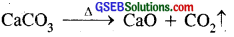The thermal stability of carbonates of alkaline earth metals increase down the group.
BeCO3 is least stable and BaCO3 is most stable.

(ii) Solubility:
All the carbonates of alkali metals are generally soluble in water and their solubility increases rapidly on descending the group. This is due to the reason that their lattice energies decrease more rapidly than their hydration energies down the group. In the case of carbonates of alkaline earth metals they are sparingly soluble in water and their solubility decreases down the group from Be to Ba. For example, MgCO3 is slightly soluble in water, but BaCO3 is almost insoluble.

(c) Sulphates of alkaline and alkaline earth metals.

(i) The sulphates of alkali metals are thermally quite stable whereas the sulphates of alkaline earth metals decompose on heating to give oxides and SO3. The temp, of decomposition increases down the group.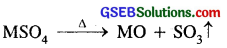(ii) The sulphates of alkaline metals Na and K are soluble in water. As far as the solubility of sulphates of alkaline earth metal in water is concerned, BeSO4 and MgSO4 are highly soluble, CaSO4 is sparingly soluble, but the sulphates of Sr, Ba and Ra are virtually insoluble. Thus the solubility of their sulphates in water decreases down the group.
BeSO4 > MgSO4 > > CaSO4 > SiSO4 > BaSO4.Question 16.
Starting with sodium chloride how would you proceed to prepare
(i) Sodium metal
(ii) Sodium hydroxide
(iii) Sodium peroxide
(iv) Sodium carbonate?
(i) Sodium metal from sodium chloride : Sodium is prepared from fused (molten) sodium chloride. Sodium chloride is mixed with CaCl2 and KF [to lower the M.Pt. of NaCl to 85O-875k] and subjected to electrolysis (in DOWN’S CELL) when the following reactions occur.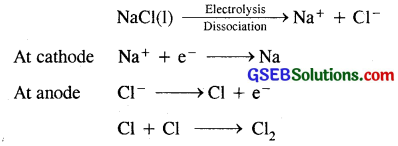Sodium, liberated at the cathode, is collected in kerosene oil chlorine gas is liberated at the anode.

(ii) Sodium hydroxide from sodium chloride : Sodium hydroxide (caustic soda) is generally prepared by the electrolysis of brine solution (NaCl solution in water) in Castner Kellner cell. A mercury cathode and carbon anode are used. Sodium metal discharged at the cathode combines with mercury to form sodium amalgam. Cl2 gas is evolved at the anode.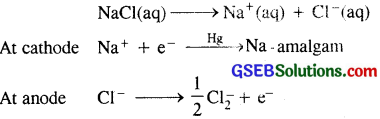The amalgam is treated with water to give sodium hydroxide and hydrogen gas.
2Na-amalgam + 2H2O → 2NaOH + 2Hg + H2

(iii) Sodium peroxide from sodium chloride : Sodium metal obtained, by the electrolysis of molten sodium chloride is heated with O2 at about 575 K when sodium forms mainly sodium peroxide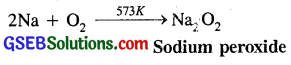(iv) Sodium carbonate (Na2CO3) from sodium chloride : Sodium carbonate is prepared from an aqueous sol if km of NaCl by SOLVAY PROCESS. In this process CO2 is passed through NaCl solution saturated with ammonia, when following reactions occur.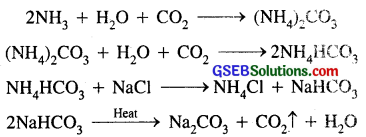Question 17.
What happens when :
(i) Magnesium is burnt in air
(ii) Quick lime is heated with silica
(iii) Chlorine reacts with slaked lime
(iv) Calcium nitrate is heated?
(i) When magnesium is burnt in air, dazzling brilliant light is given out and a mixture of magnesium oxide and magnesium nitride is formed.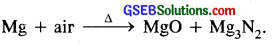(ii) Quick lime is treated with silica: When quick lime (CaO) is treated with silica (SiO2) at high temp, calcium silicate (CaSiO3) is formed.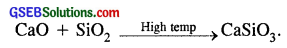(iii) Chlorine reacts with slaked lime : When Cl2 gas is passed through milk of lime (slaked lime) Ca(OH)2 it forms calcium hypochlorite, a constituent of bleaching powder.
2Ca(OH)2 + 2C2 → CaCl2 + Ca(OCl)2 + 2H2O.

(iv) Calcium nitrate is heated: When calcium nitrate is heated, it decomposes to give calcium oxide, NO2 and O2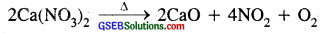Question 18.
Describe two important uses of each of the following:
(i) Caustic soda
(ii) Sodium carbonate
(iii) Quicklime.
(i) Two important uses of caustic soda :

• It is used in paper, soap and rayon and textile industries.
• It is useful in refining of petroleum.

(ii) Two important uses of sodium carbonate :

• It is used in the manufacture of glass, soap, borax and caustic soda.
• It is used in water softening, laundering and cleaning.

(iii) Two important uses of quick lime :

• It is used in the purification of sugar and in the manufacture of dye stuffs.
• It is an important primary material and is the cheapest form of alkali.Question 19.
Draw the structure of
(i) BeCl2 (vapour)
(ii) BeCl2 (solid).
(i) Structure of BeCl2 (vapour) : BeCl2 has bridged chloride structure in the vapour phase.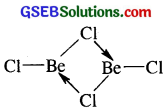(ii) Structure of BeCi2 in the solid state : BeCl2 has a chain structure in the solid state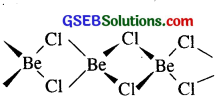Question 20.
The hydroxides and carbonates of sodium and potassium are easily soluble in water while the corresponding salts of magnesium and calcium are sparingly soluble in water. Explain.
The solubility of a salt in water depends upon their –
(i) lattice energy
(ii) hydration energy.
In the case of hydroxides and carbonates of sodium and potassium their hydration energy is more than their lattice energy. Therefore they dissolve in water. In the case of hydroxides and carbonates of magnesium and calcium their hydration energy is less than their lattice energy. Therefore they are sparingly soluble.

Question 21.
Describe the importance of the following
(i) lime stone
(ii) Cement
(iii) Plaster of paris.
(i) Importance of lime stone : Lime stone is an important primary material and is the cheapest form of alkali.

(a) It is used to prepare Quick lime CaO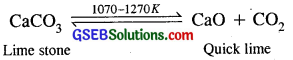(b) Quick lime when added to water in a limited amount is used to produce slaked lime or milk of lime Ca(OH)2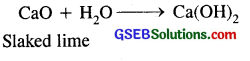(c) It is used as a flux to remove acidic impurities
CaO + SiO2 → CaSiO3
6CaO + P4O10 → 2Ca3(PO4)2

(ii) Importance of cement: Cement is an important building material. It is used in the concrete and reinforced concrete, in plastering and in construction of dams, bridges and buildings.

(iii) Importance of Plaster of Paris : Because of remarkable property of setting with water, Plaster of Paris finds use in building industry and in plasters. It is used for immobilising the affected part of the organ where their is bone fracture or sprain. It is also used in dentistry and taking casts of statues and busts.Question 22.
Why are lithium salts commonly hydratpd and those of other alkali ions usually anhydrous?
Lithium salts are commonly hydrated like LiCl.2H2O whereas other alkali ions are usually anhydrous.

The hydration enthalpy of Li+ ion is maximum among the alkali metal ions. Because of its extremely small size, Li+ ion is maximum hydrated and therefore, the effective size of Li+ in aqueous solution is the largest. The hydration enthalpy decreases with increase in ionic size
Li+ > Na+ > K+ > Rb+ > Cs+
Therefore, the ions of other alkali metals are usually anhydrous.

Question 23.
Why is LiF almost insoluble in water whereas LiCl is soluble not only in water but also acetone?
LiF is almost insoluble in water [O.27g/100g H2O] due to its high lattice energy. Due to high hydration energy of Li+ ion LiCl is soluble in water. LiCl is also soluble in acetone because of its predominantly covalent nature. With the increasing size of the anion covaleht character increases.

Question 24.
Explain the significance of sodium, potassium, magnesium and calcium in biological fluids.
Sodium and potassium : Cations play a very important role in biological fluids. They are present in biological fluids and the ratio of K to Na is 7:1 in mammals such as human beings, rats, horses. These cations accummulate in cells and create a concentration gradient and potential in the membrane. Electric pulse in the nerve is generated when a chemical is released during activation of discharge the membrane potential.

Magnesium is part of chlorophyll the green colouring matter of plants. Photosynthesis takes place only in the presence of chlorophyll.

Calcium is present in the form of calcium phosphate Ca3(PO4)2, in bones. Ca and Mg play a key role in formation of phosphorus oxygen linkages in biological systems for storage of energy. The pyrophosphate hydrolysis which releases energy is controlled by calcium ions. These ions perform important biological functions such as maintenance of ion balance and nerve impulse conduction.Question 25.
What happens when
(i) sodium metal is dropped in water
(ii) sodium metal is heated in free supply of air
(iii) sodium peroxide dissolves in water.
(i) When sodium metal is dropped in water hydrogen gas in liberated. Due to extreme heat released as the reaction is exothermic, H2 gas catches fire.
2Na + 2H2O → 2NaOH + H2

(ii) When sodium metal is heated in free supply of air or oxygen, it burns forming a mixture of sodium monoxide and sodium peroxide.
4Na + O2 → 2Na2O
2Na2O + O2 → 2Na2O2.

(iii) When sodium peroxide is dissolved in water, it gives H2O2
Na2O2 + 2H2O → 2NaOH + H2O2.

Question 26.
Comment on each of the following observations
(a) The mobilities of the alkali metal ions in aqueous solution are Li+ < Na+ < K+ < Rb+< Cs+

(b) Lithium is the only alkali metal to form a nitride directly.

(c) E° for M2+(aq) + 2e → M(s)
(where M = Ca, Sr, Ba) is nearly constant.
(a) Due to its smallest size among the alkali metal ions, Li+ has the maximum degree of hydration. Due to its maximum hydration its mobility is minimum.
As the size increases from Li+ to Cs+ the ionic size increases, hence degree of hydration decreases and hence ionic mobility increases.
∴ Mobilities of alkali metal ions in aqueous solutions follow the order
Li+ < Na+ < K+ < Rb+ < Cs+

(b) Lithium is.the only alkali metal which forms a nitride directly Li+ and N3- ions are very small, so they form stable Li3N.
6 Li + N2 → 2Li3N
Li+ ion being the smallest will combine with small N-3 ions to form a stable crystal lattice.

(c) E° for M2+ + 2e → M(s) where M = Ca, Sr, Ba is nearly same.
Be2+, Mg2+ ions are smaller in size as compared to Ca2+, Sr2+ or Ba2+.
∴ They can lose electrons readily rather than Be, Mg.
Ca → Ca2+ + 2e
or their standard reduction potentials are having mote negative values rather than Be2+or Mg2-
∵ tendency to lose electrons is almost similar
∴ E° for Ca2+, Sr2+, Ba2+ is nearly constant (- 2.89 V).

Question 27.
State as to why
(a) a solution of Na2CO3 is alkaline?
(b) alkali metals are prepared by electrolysis of their fused chlorides?
(c) sodium is found to be more useful than potassium?
(a) Na2CO3 solution is alkaline due to hydrolysis of Na2CO3. Sodium carbonate is the salt of a strong base NaOH and a weak acid H2CO3. Due to hydrolysis, the resultant solution is alkaline due to the presence of excess of OH ions
Na2CO3 + 2H2O → 2NaOH + H2CO3.

(b)

• Alkali metals are strong reducing agents and hence can’t be extracted by the reduction of their oxides or chlorides.
• Alkali metals being highly electro +ve, cannot be displaced from the aqueous solutions of their salts by other metals.
• Alkali metals cannot be isolated by the electrolysis of the aqueous solution of their salts since H2 gas is liberated at the cathode instead of the alkali metal.

(c) Sodium is more useful than potassium

• Sodium is used as a reducing agent.
• It is used in Lassaigne’s test for detection of N, S & halogens.
• About 60% of world production of sodium is used to make TEL Pb(C2H5)4 an antiknock.
• It is used in sodium vapour lamp.Question 28.
Write balanced equations for reactions between
(a) Na2O2 and water
(b) KO2 and water
(c) Na2O and CO2.
(a) Na2O2 + 2H2O → 2NaOH + H2O

(b) 4KO2 + 2H2O → 4KOH + 3O2
or 2KO2 + 2H2O → KOH + H2O2 + O2

(c) Na2O + CO2 → Na2O3.

Question 29.
How would you explain the following observations?
(i) BeO is almost insoluble but BeSO4 is soluble in water.
(ii) BaO is soluble but BaSO4 is insoluble in water.
(iii) LiI is more soluble than KI in ethanol.
(i) BeO is insoluble in water because in the case of BeO, its lattice energy is greater than its hydration energy whereas BeSO4 is soluble in water because in this case hydration energy is more than the lattice energy.

(ii) In the case of solubility of oxides of II group elements both the lattice energy and hydration energy decreases down the group as the size of cation increases, but lattice energy decreases more sharply that the hydration energy, hence BaO is soluble in water as its hydration energy exceeds its lattice energy. BaSO4, on the other hand is insoluble in water.

The magnitude of lattice energy remains almost constant as the ion is so big that the small increase in the size of the cations from Be2+ to Ba2+ does not make any difference. However the hydration energy decreases appreciably from Be2+ to Ba2+ as the size of the cation increases. Hence in the case of BaSO4, its lattice energy happens to be more than its hydration energy. Therefore BaSO4 is insoluble in water.

(iii) Lil is more soluble in ethanol than KI. In the case of Lil due to small size of Li+, greater is its polarising power and hence larger is the covalent character of its molecule Lil. Hence it is soluble in covalent solvents like ethanol. In the case of KI, it being an ionic compound, is less soluble in ethanol.

Question 30.
Which of the alkali metal is having least melting point?
(a) Na
(b) K
c) Rb
(d) Cs.
(d) Caesium is having the least melting point (= 312 K).Question 31.
Which one of the following alkaii metal gives hydrated salts?
(a) Li
(b) Na
(c) K
(d) Cs.